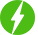# Machine Learning with Python : COMPLETE COURSE FOR BEGINNERS

## Requirements

• No prior experience needed, you will learn what is needed. (A basic python knowledge will definetly increase your chances of learning fast))

## Description

Machine Learning and artificial intelligence (AI) is everywhere; if you want to know how companies like Google, Amazon, and even Udemy extract meaning and insights from massive data sets, this data science course will give you the fundamentals you need. Data Scientists enjoy one of the top-paying jobs, with an average salary of \$120,000 according to Glassdoor and Indeed. That’s just the average! And it’s not just about money – it’s interesting work too!

Machine Learning (Complete course Overview)

Foundations

• Introduction to Machine Learning
• Intro
• Application of machine learning in different fields.
• Advantage of using Python libraries. (Python for machine learning).
• Python for AI & ML
• Python Basics
• Python functions, packages, and routines.
• Working with Data structure, arrays, vectors & data frames. (Intro Based with some examples)
• Jupyter notebook- installation & function
• Pandas, NumPy, Matplotib, Seaborn
• Applied Stastistics
• Descriptive statistics
• Probability & Conditional Probability
• Hypothesis Testing
• Inferential Statistics
• Probability distributions – Types of distribution – Binomial, Poisson & Normal distribution

Machine Learning

• Supervised Learning
• Multiple variable Linear regression
• Regression
• Introduction to Regression
• Simple linear regression
• Model Evaluation in Regression Models
• Evaluation Metrics in Regression Models
• Multiple Linear Regression
• Non-Linear Regression
• Naïve bayes classifiers
• Multiple regression
• K-NN classification
• Support vector machines
• Unsupervised Learning
• Intro to Clustering
• K-means clustering
• High-dimensional clustering
• Hierarchical clustering
• Dimension Reduction-PCA
• Classification
• Introduction to Classification
• K-Nearest Neighbours
• Evaluation Metrics in Classification
• Introduction to decision tress
• Building Decision Tress
• Into Logistic regression
• Logistic regression vs Linear Regression
• Logistic Regression training
• Support vector machine
• Ensemble Techniques
• Decision Trees
• Bagging
• Random Forests
• Boosting
• Featurization, Model selection & Tuning
• Feature engineering
• Model performance
• ML pipeline
• Grid search CV
• K fold cross-validation
• Model selection and tuning
• Regularising Linear models
• Bootstrap sampling
• Randomized search CV
• Recommendation Systems
• Introduction to recommendation systems
• Popularity based model
• Hybrid models
• Content based recommendation system
• Collaborative filtering

• EDA
• Pandas-profiling library
• Time series forecasting
• ARIMA Approach
• Model Deployment
• Kubernetes

Capstone Project

If you’ve got some programming or scripting experience, this course will teach you the techniques used by real data scientists and machine learning practitioners in the tech industry – and prepare you for a move into this hot career path.

Each concept is introduced in plain English, avoiding confusing mathematical notation and jargon. It’s then demonstrated using Python code you can experiment with and build upon, along with notes you can keep for future reference. You won’t find academic, deeply mathematical coverage of these algorithms in this course – the focus is on practical understanding and application of them. At the end, you’ll be given a final project to apply what you’ve learned!

Our Learner’s Review: Excellent course. Precise and well-organized presentation. The complete course is filled with a lot of learning not only theoretical but also practical examples. Mr. Risabh is kind enough to share his practical experiences and actual problems faced by data scientists/ML engineers. The topic of “The ethics of deep learning” is really a gold nugget that everyone must follow. Thank you, 1stMentor  and SelfCode Academy for this wonderful course.

## Who this course is for:

• Beginner Python Developers enthusiastic about Learning Machine Learning and Data Science
• Anyone interested in Machine Learning.
• Students who have at least high school knowledge in math and who want to start learning Machine Learning.
• Any intermediate level people who know the basics of machine learning, including the classical algorithms like linear regression or logistic regression, but who want to learn more about it and explore all the different fields of Machine Learning.
• Any people who are not that comfortable with coding but who are interested in Machine Learning and want to apply it easily on datasets.
• Any students in college who want to start a career in Data Science.
• Any data analysts who want to level up in Machine Learning.
• Any people who want to create added value to their business by using powerful Machine Learning tools.#### online educate

We will be happy to hear your thoughts